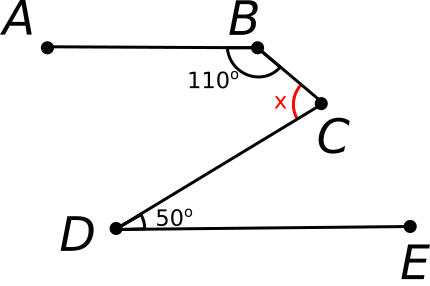# Angles in a Snakey Segment

Geometry Level 1If $\overline{AB}$ is parallel to $\overline{DE}$, then what is the measure of angle $BCD$ in degrees?

×